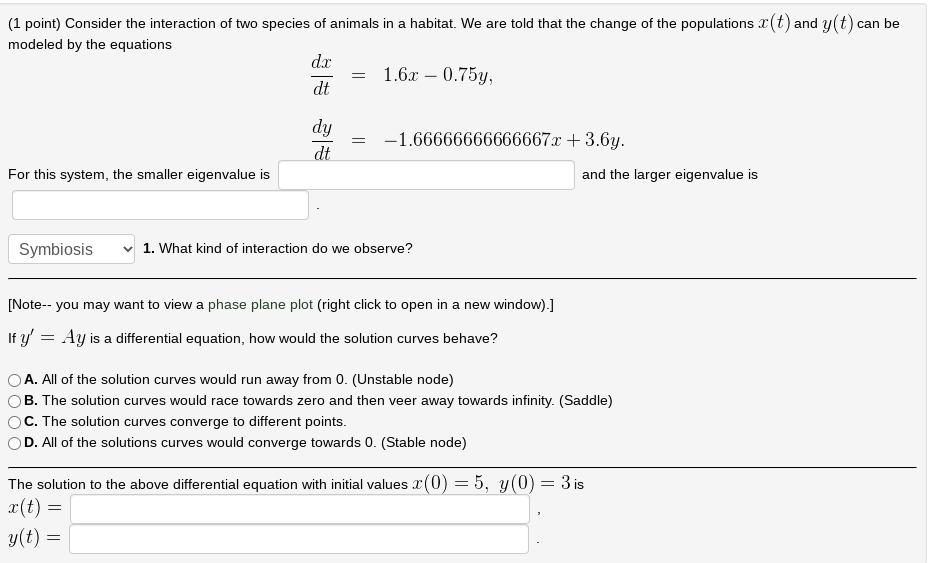# Question can be (1 point) Consider the interaction of two species of animals in a habitat. We are told that the change of the populations r(t) and y(t) modeled by the equations dx 1.6r – 0.75y, dt = dy dt = -1.66666666666667x +3.6y. For this system, the smaller eigenvalue is and the larger eigenvalue is Symbiosis 1. What kind of interaction do we observe? [Note-- you may want to view a phase plane plot (right click to open in a new window).] If y' = Ay is a differential equation, how would the solution curves behave? — A. All of the solution curves would run away from 0. (Unstable node) B. The solution curves would race towards zero and then veer away towards infinity. (Saddle) C. The solution curves converge to different points. D. All of the solutions curves would converge towards 0. (Stable node) = = The solution to the above differential equation with initial values x(0) = 5, y(0) = 3 is x(t) = g(t) = = =